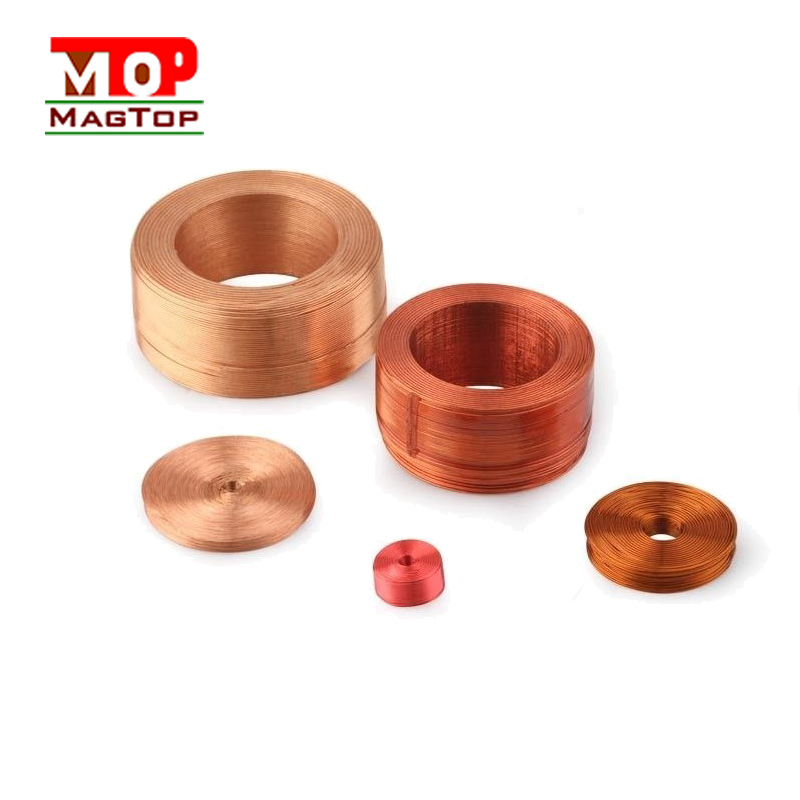# What is the purpose of the coil

##### author: MagTop
07/02/2023Company News | common mode chokes | Industry News | transformer|RJ45 connectors
Coil functions are: choke action, tuning frequency action, magnetic field generation, refers to the inductor.
Choke action: the induction coil in the induction electromotive force is always against the current change in the coil, the induction coil has a blocking effect on the AC current, the size of the blocking effect is called inductance xl, the unit is ohm, the inductance l and the AC frequency f relationship is xl=2πfl, inductors are mainly divided into high frequency choke and low frequency choke.
Tuning frequency action:
Through parallel inductors and capacitors, lc tuned circuit can be formed, that is, when the natural vibration frequency of the circuit f0 and the frequency of non-AC signal f are equal, the inductance and capacitance of the circuit are also equal, and the electromagnetic energy goes back and forth between the inductance and capacitance, resulting in the resonance phenomenon of the lc circuit. When resonant, the inductance of the circuit is equivalent to the capacitive impedance, reverse.
The overall current inductance of the circuit is minimum, and the current flow is maximum (refers to the AC signal f= "f0"). lc resonant circuit has the function of selecting frequency to select the AC signal of a certain frequency f.
Generate a magnetic field: The coil also produces a magnetic field, which uses the principle of electromagnetic induction to turn its engine.
Refers to the inductor: coil is wound one by one, insulated each other, but the insulated tube can be hollow, can also contain iron core or magnetic powder core, referred to as inductance, inductance is divided into fixed inductance and variable inductor, fixed inductance winding referred to as inductance or winding.